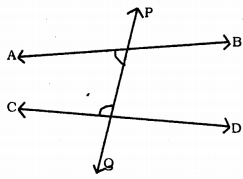# KSEEB Solutions for Class 9 Maths Chapter 2 Introduction to Euclid Geometry Ex 2.2

KSEEB Solutions for Class 9 Maths Chapter 2 Introduction to Euclid Geometry Ex 2.2 are part of KSEEB Solutions for Class 9 Maths. Here we have given Karnataka Board Class 9 Maths Chapter 2 Introduction to Euclid Geometry Exercise 2.2.

## Karnataka Board Class 9 Maths Chapter 2 Introduction to Euclid Geometry Ex 2.2

Question 1.
How would you rewrite Euclid’s fifth postulate so that it would be easier to understand?
Euclid’s fifth postulate states that “If a straight line falling on two straight lines makes the interior angles on the same side of it taken together less than two right angles, then the two straight lines, if produced indefinitely meet on that side on which the sum of angles is less than two right angles.”For example, the line PQ falls on lines AB and CD such that the sum of the interior angles 1 and 2 is less than 180° on the left side of PQ. Therefore, the lines AB and CD will eventually intersect on the left side of PQ.
In the above figure, ∠APQ + ∠PQC < 180° then AB and CD meet at the side of A and C.

Question 2.
Does Euclid’s fifth postulate imply the existence of parallel lines ? Explain.